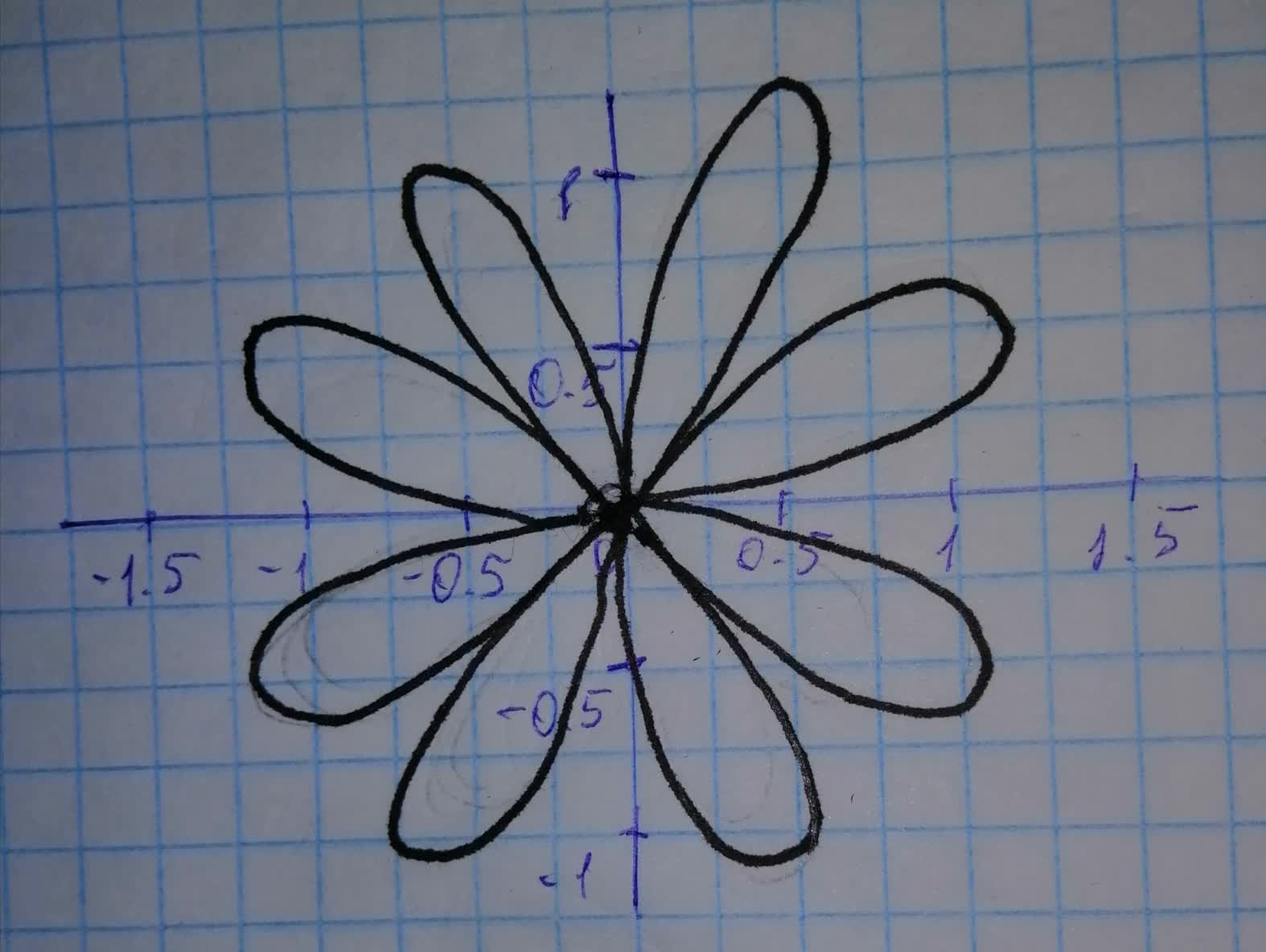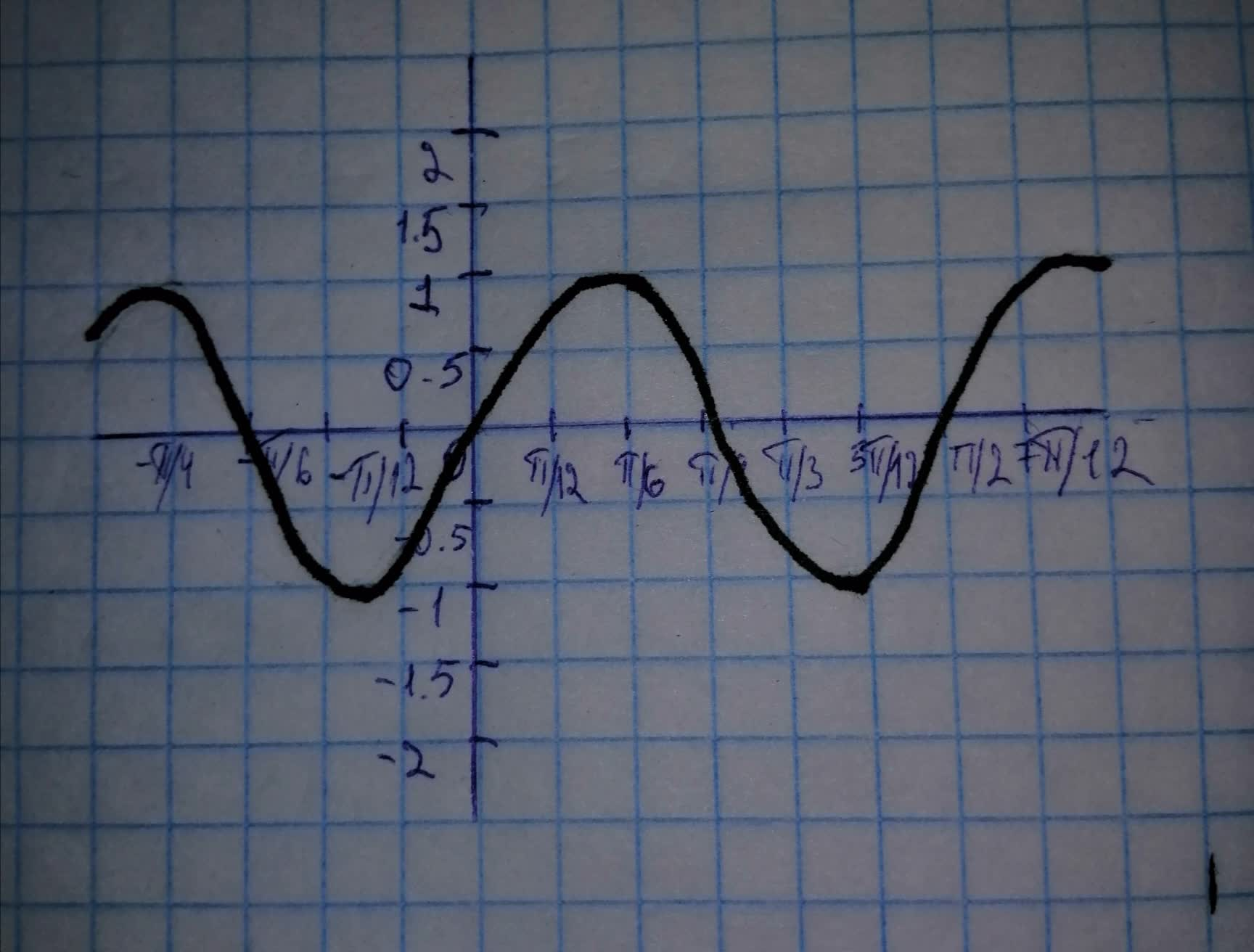# Find the area of the region enclosed by one loop of the curve. r=\sin 4 \thetuneskovogl5 2021-11-16 Answered
Find the area of the region enclosed by one loop of the curve. $r=\mathrm{sin}4\theta$
You can still ask an expert for help

• Questions are typically answered in as fast as 30 minutes

Solve your problem for the price of one coffee

• Math expert for every subject
• Pay only if we can solve itAnnie Midgett

Step 1
$r=\mathrm{sin}\left(4\theta \right)$
Area enclosed by one of the loops will be simply
${\int }_{a}^{b}\frac{{r}^{2}}{2}d\theta$
We will find the limits of integration a, b, and then we will integrate the integral to find the area enclosed by one of the loops.Step 2
To find the limits of integration, we need to find two consecutive values of $\theta$ for which r is zero.
Graph of $y=\mathrm{sin}4x$ is shown below:
We can see that 0 and $\frac{\pi }{4}$ are two consecutive values for which $\mathrm{sin}4x$ is zero
Therefore, we can integrate from 0 to $\frac{\pi }{4}$
You can also integrate from $-\frac{\pi }{4}$ to 0
The only condition is that you have to integrate between two consecutive values of $\theta$ for which $r=0$Step 3
Therefore, the limit of integration is from 0 to $\frac{\pi }{4}$
Area enclosed by one of the loops is
${\int }_{0}^{\frac{\pi }{4}}\frac{{r}^{2}}{2}d\theta$
${\int }_{0}^{\frac{\pi }{4}}\frac{{\left(\mathrm{sin}\left(4\theta \right)\right)}^{2}}{2}d\theta$
${\int }_{0}^{\frac{\pi }{4}}\frac{{\mathrm{sin}}^{2}\left(4\theta \right)}{2}d\theta$
${\int }_{0}^{\frac{\pi }{4}}\left(\frac{1-\mathrm{cos}\left(8\theta \right)}{4}\le ft\right)d\theta$
We used the formula ${\mathrm{sin}}^{2}\theta =\frac{1-\mathrm{cos}2\theta }{2}$
${\left[\frac{\theta }{4}-\frac{\mathrm{sin}\left(8\theta \right)}{32}\right]}_{0}^{\frac{\pi }{4}}=\frac{\pi }{16}$
Area enclosed by the loop is $\frac{\pi }{16}$
###### Not exactly what you’re looking for?Philip O'Neill

Step 1
The given curve is $r=\mathrm{sin}4\theta$
Area of the curve enclosed in the first loop is,
$A={\int }_{a}^{b}\frac{1}{2}{r}^{2}d\theta$
Step 2
Two consecutive value of theta for which $\mathrm{sin}4\theta$,
$\mathrm{sin}4\theta =0$

Step 3
Now integrate from 0 to $\frac{\pi }{4}$ .
Area enclosed by one of the loop is,
$A={\int }_{0}^{\frac{\pi }{4}}\frac{1}{2}{\left(\mathrm{sin}4\theta \right)}^{2}d\theta$
$=\frac{1}{2}{\int }_{0}^{\frac{\pi }{4}}\left(\frac{1-\mathrm{cos}8\theta }{2}\right)d\theta \left[\mathrm{cos}2\theta =1-2{\mathrm{sin}}^{2}\theta \right]$
$=\frac{1}{4}{\int }_{0}^{\frac{\pi }{4}}\left(1-\mathrm{cos}8\theta \right)d\theta$
$=\frac{1}{4}{\int }_{0}^{\frac{\pi }{4}}\left(1\right)d\theta -\frac{1}{4}{\int }_{0}^{\frac{\pi }{4}}\left(\mathrm{cos}8\theta \right)d\theta$
$=\frac{1}{4}\left({\left[\theta \right]}_{0}^{\frac{\pi }{4}}-{\left[\frac{\mathrm{sin}8\theta }{8}\right]}_{0}^{\frac{\pi }{4}}\right)$
$=\frac{1}{4}\left(\left(\frac{\pi }{4}-0\right)-\left(\frac{\mathrm{sin}2\pi -\mathrm{sin}0}{8}\right)\right)$
$=\frac{1}{4}\left(\frac{\pi }{4}-\left(\frac{0-0}{8}\right)\right)$
$=\frac{\pi }{16}$